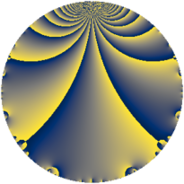# Properties

 Label 8016.2.a.kLevel $8016$ Weight $2$ Character orbit 8016.a Self dual yes Analytic conductor $64.008$ Analytic rank $0$ Dimension $2$ CM no Inner twists $1$

# Related objects

## Newspace parameters

 Level: $$N$$ $$=$$ $$8016 = 2^{4} \cdot 3 \cdot 167$$ Weight: $$k$$ $$=$$ $$2$$ Character orbit: $$[\chi]$$ $$=$$ 8016.a (trivial)

## Newform invariants

 Self dual: yes Analytic conductor: $$64.0080822603$$ Analytic rank: $$0$$ Dimension: $$2$$ Coefficient field: $$\Q(\sqrt{2})$$ Defining polynomial: $$x^{2} - 2$$ Coefficient ring: $$\Z[a_1, \ldots, a_{5}]$$ Coefficient ring index: $$1$$ Twist minimal: no (minimal twist has level 1002) Fricke sign: $$-1$$ Sato-Tate group: $\mathrm{SU}(2)$

## $q$-expansion

Coefficients of the $$q$$-expansion are expressed in terms of $$\beta = \sqrt{2}$$. We also show the integral $$q$$-expansion of the trace form.

 $$f(q)$$ $$=$$ $$q + q^{3} + ( -2 + \beta ) q^{5} + 2 \beta q^{7} + q^{9} +O(q^{10})$$ $$q + q^{3} + ( -2 + \beta ) q^{5} + 2 \beta q^{7} + q^{9} -2 q^{11} + 2 \beta q^{13} + ( -2 + \beta ) q^{15} + ( -4 + \beta ) q^{17} + ( -4 - 2 \beta ) q^{19} + 2 \beta q^{21} + 4 \beta q^{23} + ( 1 - 4 \beta ) q^{25} + q^{27} + 2 q^{29} + ( -4 + 2 \beta ) q^{31} -2 q^{33} + ( 4 - 4 \beta ) q^{35} -4 q^{37} + 2 \beta q^{39} + ( -4 + \beta ) q^{41} + ( 4 + 3 \beta ) q^{43} + ( -2 + \beta ) q^{45} + ( 10 - 2 \beta ) q^{47} + q^{49} + ( -4 + \beta ) q^{51} + ( -2 + 3 \beta ) q^{53} + ( 4 - 2 \beta ) q^{55} + ( -4 - 2 \beta ) q^{57} + ( 10 + 2 \beta ) q^{59} + 10 \beta q^{61} + 2 \beta q^{63} + ( 4 - 4 \beta ) q^{65} -\beta q^{67} + 4 \beta q^{69} + ( 4 + 6 \beta ) q^{71} + ( -2 + 10 \beta ) q^{73} + ( 1 - 4 \beta ) q^{75} -4 \beta q^{77} + ( 6 + 3 \beta ) q^{79} + q^{81} + ( 6 + 4 \beta ) q^{83} + ( 10 - 6 \beta ) q^{85} + 2 q^{87} + ( -2 - 8 \beta ) q^{89} + 8 q^{91} + ( -4 + 2 \beta ) q^{93} + 4 q^{95} + ( -6 - 8 \beta ) q^{97} -2 q^{99} +O(q^{100})$$ $$\operatorname{Tr}(f)(q)$$ $$=$$ $$2q + 2q^{3} - 4q^{5} + 2q^{9} + O(q^{10})$$ $$2q + 2q^{3} - 4q^{5} + 2q^{9} - 4q^{11} - 4q^{15} - 8q^{17} - 8q^{19} + 2q^{25} + 2q^{27} + 4q^{29} - 8q^{31} - 4q^{33} + 8q^{35} - 8q^{37} - 8q^{41} + 8q^{43} - 4q^{45} + 20q^{47} + 2q^{49} - 8q^{51} - 4q^{53} + 8q^{55} - 8q^{57} + 20q^{59} + 8q^{65} + 8q^{71} - 4q^{73} + 2q^{75} + 12q^{79} + 2q^{81} + 12q^{83} + 20q^{85} + 4q^{87} - 4q^{89} + 16q^{91} - 8q^{93} + 8q^{95} - 12q^{97} - 4q^{99} + O(q^{100})$$

## Embeddings

For each embedding $$\iota_m$$ of the coefficient field, the values $$\iota_m(a_n)$$ are shown below.

For more information on an embedded modular form you can click on its label.

Label $$\iota_m(\nu)$$ $$a_{2}$$ $$a_{3}$$ $$a_{4}$$ $$a_{5}$$ $$a_{6}$$ $$a_{7}$$ $$a_{8}$$ $$a_{9}$$ $$a_{10}$$
1.1
 −1.41421 1.41421
0 1.00000 0 −3.41421 0 −2.82843 0 1.00000 0
1.2 0 1.00000 0 −0.585786 0 2.82843 0 1.00000 0
 $$n$$: e.g. 2-40 or 990-1000 Significant digits: Format: Complex embeddings Normalized embeddings Satake parameters Satake angles

## Atkin-Lehner signs

$$p$$ Sign
$$2$$ $$-1$$
$$3$$ $$-1$$
$$167$$ $$-1$$

## Inner twists

This newform does not admit any (nontrivial) inner twists.

## Twists

By twisting character orbit
Char Parity Ord Mult Type Twist Min Dim
1.a even 1 1 trivial 8016.2.a.k 2
4.b odd 2 1 1002.2.a.f 2
12.b even 2 1 3006.2.a.n 2

By twisted newform orbit
Twist Min Dim Char Parity Ord Mult Type
1002.2.a.f 2 4.b odd 2 1
3006.2.a.n 2 12.b even 2 1
8016.2.a.k 2 1.a even 1 1 trivial

## Hecke kernels

This newform subspace can be constructed as the intersection of the kernels of the following linear operators acting on $$S_{2}^{\mathrm{new}}(\Gamma_0(8016))$$:

 $$T_{5}^{2} + 4 T_{5} + 2$$ $$T_{7}^{2} - 8$$ $$T_{11} + 2$$ $$T_{13}^{2} - 8$$

## Hecke characteristic polynomials

$p$ $F_p(T)$
$2$ $$T^{2}$$
$3$ $$( -1 + T )^{2}$$
$5$ $$2 + 4 T + T^{2}$$
$7$ $$-8 + T^{2}$$
$11$ $$( 2 + T )^{2}$$
$13$ $$-8 + T^{2}$$
$17$ $$14 + 8 T + T^{2}$$
$19$ $$8 + 8 T + T^{2}$$
$23$ $$-32 + T^{2}$$
$29$ $$( -2 + T )^{2}$$
$31$ $$8 + 8 T + T^{2}$$
$37$ $$( 4 + T )^{2}$$
$41$ $$14 + 8 T + T^{2}$$
$43$ $$-2 - 8 T + T^{2}$$
$47$ $$92 - 20 T + T^{2}$$
$53$ $$-14 + 4 T + T^{2}$$
$59$ $$92 - 20 T + T^{2}$$
$61$ $$-200 + T^{2}$$
$67$ $$-2 + T^{2}$$
$71$ $$-56 - 8 T + T^{2}$$
$73$ $$-196 + 4 T + T^{2}$$
$79$ $$18 - 12 T + T^{2}$$
$83$ $$4 - 12 T + T^{2}$$
$89$ $$-124 + 4 T + T^{2}$$
$97$ $$-92 + 12 T + T^{2}$$